# What is a cube of 3

What is a dice?
 ...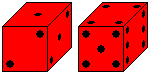A dice has 21 points or eyes. They are used to form the numbers from 1 to 6 on the side surfaces. - You roll the dice out of your hand or with a leather cup. You turn the leather cup over or let the cube roll out.
Which of the numbers 1 to 6 appears is up to chance. Therein lies the attraction of throwing the dice.
The cube is used in many board games. He often determines the course of the game. He gives a game unexpected twists that liven it up.

There are 30 dice Top

 ...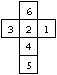... If you enter the numbers 1, 2, 3, 4, 5 and 6 and form all rearrangements (permutations) of the six numbers, you get 1 * 2 * 3 * 4 * 5 * 6 = 6! = 720 cubes. But now the cubes are also listed, which can be converted into one another by rotating around one of the 13 axes. There are 24 spins of this type. So there are only 720: 24 = 30 different dice.
The following picture explains this fact. (The numbers below the figures indicate the number of rotations.)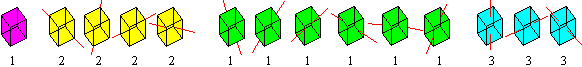Appearance of the standard cube Top
There are 30 dice. Each cube has a mirror cube in which the numbers to the right and left of the one are exchanged.

 ...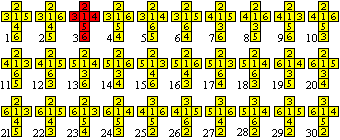... The dice can be identified by a six-digit number: You start with 1, go up, then counter-clockwise around the 1 and add the opposite number to the 1. - For example, the red cube has the representation 123546. The cubes on the left are arranged according to the size of these numbers.
You can find out more about the number 30 on my website Macmahon's Color Cubes.

In the western world, only the red dice is common.

 ...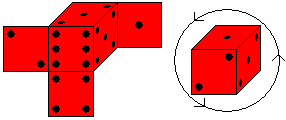... This dice has two properties:> The numbers on opposite faces add up to 7.> If you look at a corner of the cube with the numbers 1, 2 and 3, they are arranged counterclockwise.
Most dice makers adhere to these rules.

 ...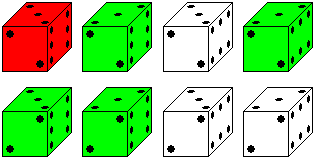... Even so, cubes can still look different.For the numbers 2, 3 and 6 there are two orientations of the eyes, which merge into each other by turning them through 180 °. That leads to eight images.The most common is the red cube in the top left, but I was also able to spot the green cube among my 20 cubes.

Following four puzzles to the dice.

Guess the sum Top

 ...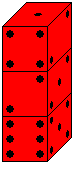... You build a tower from several cubes.Is it possible to find the sum of the eyes of the invisible horizontal cube faces?In this case the tower consists of three cubes. Five faces of the cube, namely the underside of the upper cube and the upper and lower sides of the two lower cubes must be recorded.
solution
You only pay attention to the number 1 at the top and count the dice below. The one is opposite the six. For each die you count 7. The total is 6 + 2x7 = 20.

Cube puzzle Top

 ...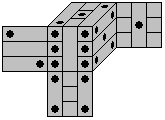... You create a nice cube puzzle when you divide the game cube into nine bars.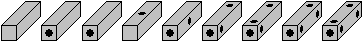In a middle layer, the bars are placed vertically and horizontally on the outside. Then the puzzle is more interesting.
 ...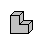... The 3x3x3 cube can also be formed from 9 V triominos (picture) or from soma cubes and assigned appropriate points.

Rubik's DiceTop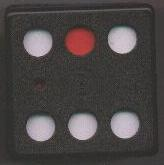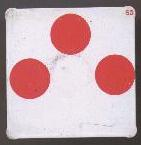Rubik's Dice is a black plastic cube 7cm x 7cm x 7cm, which instead of eyes has circular openings like a cube. In the cube there are seven white, square plates with red circles that can be placed on the inside of the walls. The plate directly at the opening will stick. You can loosen it from the outside with the supplied stamp. The plates close the openings red or white. A solution to the puzzle is found when the panels are sorted inside in such a way that the cube only has white eyes at the end.

You soon realize that you cannot solve the puzzle by trying. With seven panels there are too many ways to close the openings with the panels. Moving the plates is also troublesome.

This is how it works: You can look into the cube through the holes and draw the patterns on both sides of the plate. The plates show two-digit numbers (meaning?) At the top right that can be used as names. The result is the following picture: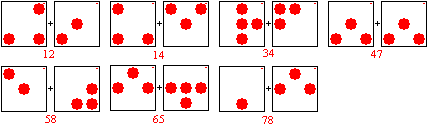Now the solution is simple. If you don't want to have fun with the solution, take action yourself now ...

Only plate 78 (left) fits on the six, then only 65 (left) on the five, then only 34 on the left on the four, then only 58 (right) on the three, then only 12 (right) on the two and then only 14 (left) on the one. The plate 47 is unnecessary.
So there is exactly one solution.

Incidentally, I've now sawed the cube :-( to scan the side of a plate. I was also curious to find out why the plates stick. - The plates are made of sheet iron. They are held by six ring-shaped magnets. These are located inside in the middle, their outlines can be seen from the outside.

Sum problem Top

 ...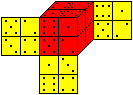... There are 8 dice. You should build a 2x2x2 cube so that the sum of the numbers on each side is the same.One of 20,736 solutions follows for the sum 14.
You can find more in the article "Dice games in the Intercity" by Reinhard Droste in 3-1980.

More cube shapes Top
The cube belongs to the five platonic solids. These are the bodies that are only formed by regular polygons. They are called tetrahedron, hexahedron (cube), octahedron, icosahedron and pentagon dodecahedron.
You can build cubes based on their model.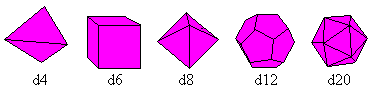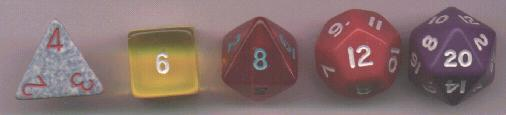They can be used to roll 4, 6, 8, 12 or 20 numbers. The number that is on top always counts. The tetrahedron is an exception. The number that is hidden counts, or rather the number that is at the top as shown in the picture.

On the internet I found other interesting cube bodies that are mathematically possible. They are convex, are formed by congruent polygons and each polygon has the same center of gravity ("Properties of Dice", URL below).

There are numerous other cube shapes. Two more are singled out.
 ...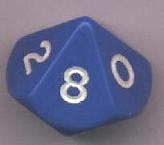... The d10 die is interesting. It has approximately the shape of a double pyramid, with the common base being roughly a pentagon. It bears the numbers 0 to 9, i.e. the digits of the tens system. In this way you can find multi-digit random numbers with several dice of this type.

 ....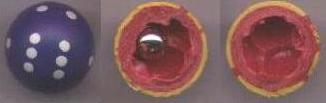... The spherical cube is original:Inside there is a steel ball that can only come to rest in one of the six hollows.Despite the spherical shape, eyes 1 to 6 always appear on top.

 ...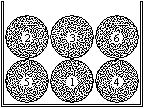... There are many ways to get random numbers. Following the example of the lottery drawing, 6 identical balls (table tennis balls!) Could be numbered, put in a container ("urn"), mixed well and then drawn one ball face down. The drawn ball bears the "rolled" number.
Rolling the dice again means to put back the drawn ball before each "dice" and only then to move it again.
This is the usual model in mathematics to simulate the cube and to integrate it into a theory.

Despite the inflation of dice shapes: The normal d6 is and will probably remain the standard dice.
It has a number of advantages:
> The number 6 is neither too big nor too small. You can play well with 6 random numbers.
> The normal cube rolls best, especially when the corners are rounded. This is common with normal dice.
> It is easy to manufacture precisely because the cube faces are perpendicular to each other.

Fake dice Top
One calls a die that shows certain eyes more often than others when thrown, a forged or marked die.

You can manipulate the normal cube in different ways:
> A die does not have six different numbers. Up to three numbers can be missing because you only ever see three sides of the cube.
> You can hardly noticeably change the outer shape of a cube. The corners can be rounded to different degrees, the side squares can be of different sizes.
> A cube can be manipulated inside. If there is an asymmetrical metal body inside, the cube will preferably move so that the heavier body is at the bottom. - A cavity will mostly be on top.
> A square area of ​​a cube can be treated in such a way that the cube prefers to rest on it when it is rolled out.
> You can use magnetic forces for manipulation, which can even be switched on by radio contact.

The boundaries between a fair and a fake die are fluid. Today, the decision is made using the chi-square test, in which typical deviations from the uniform distribution are recorded and assessed.

The computer rolls the dice Top
You can simulate rolling the dice with the computer. There is also a random generator in all programming languages ​​(e.g. in Visual Basic) that supplies decimal numbers between 0 and 1 (A = RND).

 ...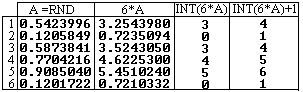... You multiply these numbers by 6, cut off the decimals with the INT function and add 1.This is demonstrated using six examples on the left.You get the numbers 4, 1, 4, 5, 6 and 1 at random.

It doesn't take much effort to "roll" the dice 6000 times.

 ...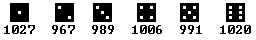... The frequency table shows that the numbers from 1 to 6 appear about the same number of times, namely about 1000x.
This is what probability theory also says. The probability of rolling a number (e.g. a three) is equal to 1/6 (= 1000: 6000). According to the law of large numbers, 1/6 is always better, the greater the number of throws. - On the other hand, a good cube simulator must have a certain degree of dispersion. This is precisely where the difficulty lies in programming a good random number generator.

A probability statement like 1/6 is not very useful for practical rolling of the dice. You don't know anything about the next litter. Each throw is independent of the previous throw.

Game dice on the internet Top

German

Faroul
cube

Wikipedia
Game dice

English

Kevin Cook
Collection of Dice

Eric W. Weisstein (MathWorld)
Dice

Klaus Æ. Mogensen
Properties of Dice

Leo van der Heijdt
Welcome to the Homepage of DICE Collector (Dutch / English)

Wikipedia
Dice

credentials Top
(1) Robert E. Lembke, Michael Schiff: The large house and family book of the games, Frankfurt a.M. 1970
(2) Martin Gardner: Mathematische Hexereien, Berlin 1988
(3) Rüdiger Thiele, Konrad Haase: The Enchanted Room, Leipzig 1991
(4) Reiner Knizia: The big book of dice games (124 games), Munich 2000

Feedback: Email address on my main page

This page is also available in German.

URL of my homepage:
http://www.mathematische-basteleien.de/

© 2001 Jürgen Köller

Top